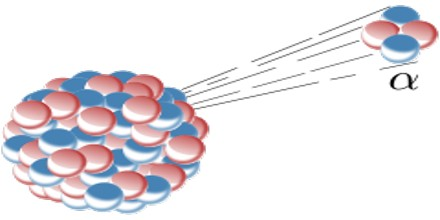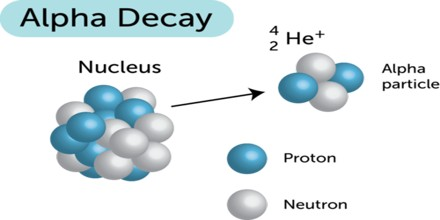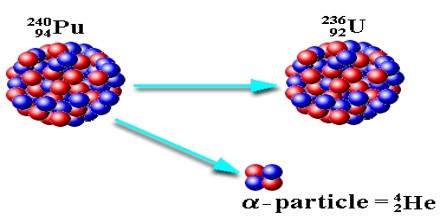Definition

Alpha decay (α decay) is the phenomenon of emission of an alpha particle from a radioactive nucleus. When a nucleus emits an alpha particle then its mass number decreases by 4 and the charge number is decreases by 2.

Alpha decay is a type of Radioactive decay is a spontaneous process in which an atomic nucleus is converted into a small nucleus with releasing of energy. This is shown by unstable radioactive nuclei that possess a less amount of binding energy for binding nucleus together. Their isotopes of radioactive compounds are also converted and get stabilize the condition.Alpha decay typically occurs in the heaviest nuclides. Theoretically, it can occur only in nuclei somewhat heavier than nickel, where the overall binding energy per nucleon is no longer a minimum and the nuclides are therefore unstable toward spontaneous fission-type processes. In practice, this mode of decay has only been observed in nuclides considerably heavier than nickel, with the lightest known alpha emitters being the lightest isotopes of tellurium.

In the alpha decay of a nucleus, the change in binding energy appears as the kinetic energy of the alpha particle and the daughter nucleus. Because this energy must be shared between these two particles, and because the alpha particle and daughter nucleus must have equal and opposite momenta, the emitted alpha particle and recoiling nucleus will each have a well-defined energy after the decay. Because of its smaller mass, most of the kinetic energy goes to the alpha particle.

Alpha Particle Decay

The Alpha particle decay is the phenomenon where by an unstable nuclei shifts to stable state by emitting an alpha particle. The Alpha particle is identical to the helium nucleus with two protons and two neutrons and sometime it is represented as He2+.Mechanism of Alpha Decay

An alpha particle is a helium-4 nucleus. This is a very stable entity and alpha emission was, historically, the first decay process to be studied in detail. Almost all naturally occurring alpha emitters are heavy elements with Z > 82. This is the only way a heavy nucleus can reduce its nucleon number, A.

The nuclear force is also short range, dropping quickly in strength beyond about 1 femtometre, while the electromagnetic force has unlimited range. The strength of the attractive nuclear force keeping a nucleus together is thus proportional to the number of nucleons, but the total disruptive electromagnetic force trying to break the nucleus apart is roughly proportional to the square of its atomic number. A nucleus with 210 or more nucleons is so large that the strong nuclear force holding it together can just barely counterbalance the electromagnetic repulsion between the protons it contains. Alpha decay occurs in such nuclei as a means of increasing stability by reducing size.Uses of Alpha Decay

Alpha decay can provide a safe power source for radioisotope thermoelectric generators used for space probes and were used for artificial heart pacemakers. Alpha decay is much more easily shielded against than other forms of radioactive decay.

Alpha particles are most commonly used in smoke alarms. These alarms contain a tiny amount of decaying Americium between two sheets of metal. The decaying Americium emits alpha radiation. A small electric current is then passed through one of the sheets and into the second one.

When the field of alpha radiation is blocked by smoke, the alarm goes off. This alpha radiation is not harmful because it is very localised and any radiation that might escape would be stopped quickly in the air.Alpha Decay Equation and Example

Alpha Decay is the process in which nuclei of the nuclide or the particle emits alpha particles or alpha rays. These emissions can be shown with the help of alpha decay equations.

Alpha Decay equation for the Uranium ”U” is shown below and further explained in detail.

238U92 —> 234Th90 + 4He2

which can also be written as:

238U92 —> 234Th90 + α

Where α is the alpha particle.

Below are given alpha decay example:

The first example is the unstable seaborgium. The seaborgium atom u nderwent a radioactive decay and in the process converted to the rutherfordium by emitting an alpha particle as seen in the equation below:

263Sg106 –> 259Rf104 + α

Here compare the difference in the atomic number and mass number of the seaborgium (Sg) and rutherfordium (Rf) is two and four respectively. The difference is due to the fact that the radioactive decay emits an alpha particle which has atomic mass and mass number of 2 and 4 respectively. So in the process the total number of sub atomic particles is conserved.